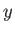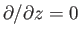Next: Complex Functions Up: Two-Dimensional Potential Flow Previous: Two-Dimensional Potential Flow

# Introduction

This chapter describes the use of complex analysis to facilitate calculations in two-dimensional, incompressible, irrotational fluid dynamics. Incidentally, incompressible, irrotational flow is usually referred to as potential flow, because the associated velocity field can be represented in terms of a velocity potential that satisfies Laplace's equation. (See Section 4.15.) In the following, all flow patterns are assumed to be such that the-coordinate is ignorable. In other words, the fluid velocity is everywhere parallel to the-plane, and. It follows that all line sources and vortex filaments run parallel to the-axis. Moreover, all solid surfaces are of infinite extent in the-direction, and have uniform cross-sections. Hence, it is only necessary to specify the locations of line sources, vortex filaments, and solid surfaces in the-plane. More information on the use of complex analysis in two-dimensional fluid mechanics can be found in Batchelor 2000, Milne-Thomson 1958, Milne-Thomson 2011, and Lamb 1993.

Richard Fitzpatrick 2016-03-31